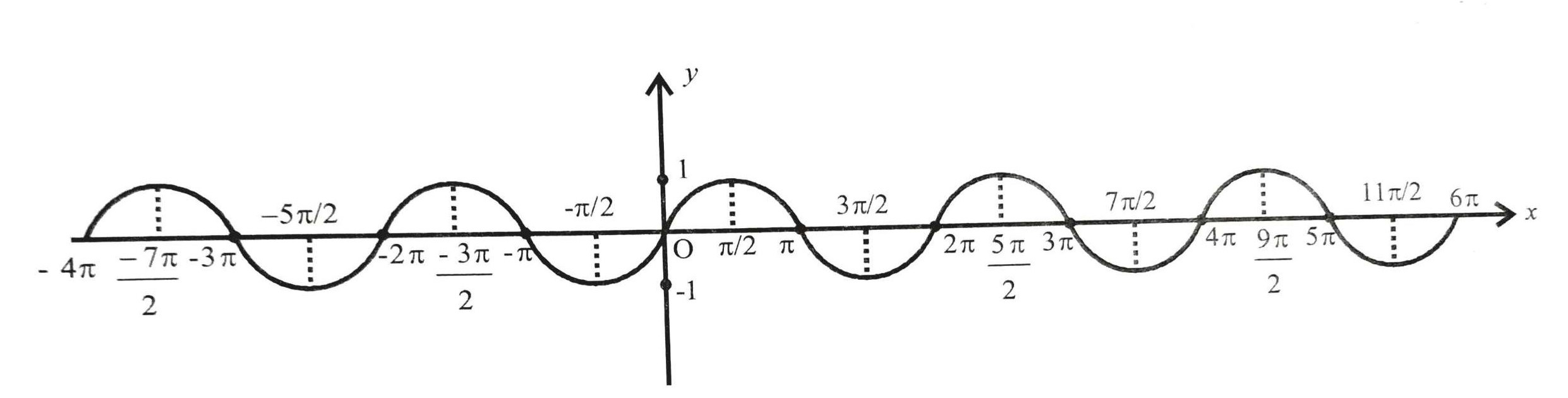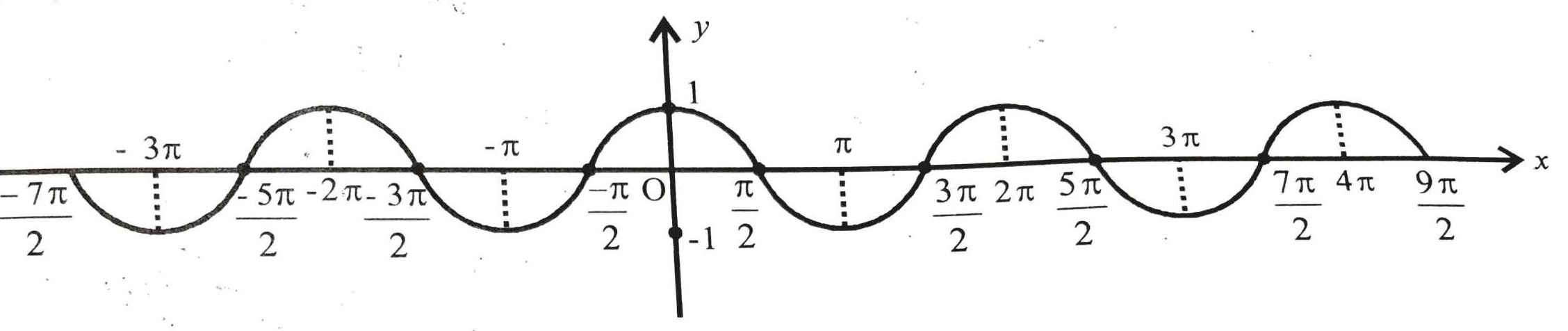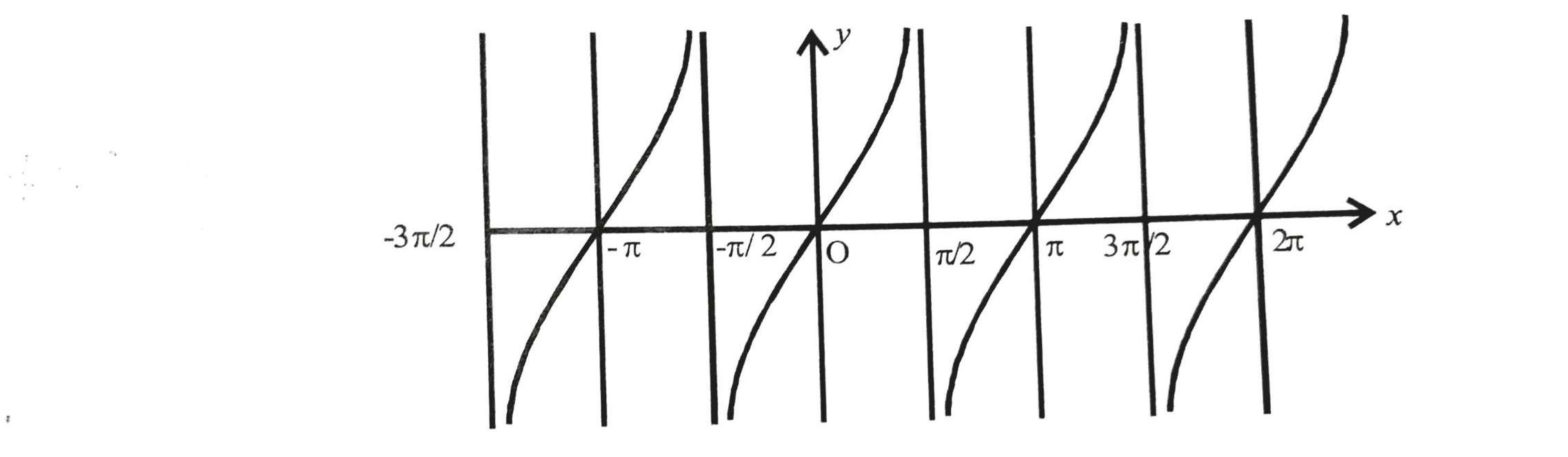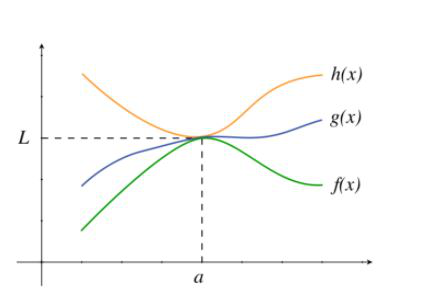Open in App
Not now

# Limits of Trigonometric Functions | Class 11 Maths

• Difficulty Level : Basic
• Last Updated : 24 Nov, 2020

There are six trigonometric functions as we know. The limit of each function tending to a point can be calculated according to the function’s continuity considering its domain and range. We can observe this in the graph of every function that the limit of each function at the same point is different.

### Sine Function

The function f(x) = sin(x) is a continuous function over its entire domain, with its domain consisting of all the real numbers. The range of this function is [-1, 1] as can be seen in the graph below:So, if the limit of sine function is calculated at any given real number it’s always defined and lies between [-1, 1].

f(x) = sin(x)

limx→a f(x) = limx→a sin(x)

= sin(a), where a is a real number

Example 1: Find limx→π/2 sin(x)

Solution:

As we know sin(x) is defined at π/2

Therefore,

limx→π/2 sin(x) = sin(π/2) = 1

Example 2: Find limx→πsin(x)

Solution:

As we know sin(x) is defined at π

Therefore,

limx→π sin(x) = sin(π) = 0

### Cosine Function

The function f(x) = cos(x) is a continuous function over its entire domain, with its domain consisting of all the real numbers.

The range of this function is [-1, 1] as can be seen in the graph below:So, if the limit of the cosine function is calculated at any given real number it’s always defined and lies between [-1, 1].

f(x) = cos(x)

limx→a f(x) = limx→a cos(x)

= cos(a), where a is a real number

Example 1: Find limx→π/2 cos(x)

Solution:

As we know cos(x) is continuous and defined at π/2

Therefore,

limx→π/2 cos(x) = cos(π/2) = 0

Example 2: Find limx→π cos(x)

Solution:

As we know cos(x) is continuous and defined at π

Therefore,

limx→π cos(x) = cos(π) = -1

### Tangent Function

The function f(x) = tan(x) is defined at all real numbers except the values where cos(x) is equal to 0, that is, the values π/2 + πn for all integers n. Thus, its domain is all real numbers except π/2 + πn, n € Z.

The range of this function is (-∞, +c).So, if the limit of the tangent function is calculated in its domain it always defined and lies between(-∞, +∞).

f(x) = tan(x)

limx→a f(x) = limx→a  tan(x)

= tan(a), where a belongs to real no. except π/2 + πn, n € Z

Example 1: Find limx→π/2tan(x)

Solution:

As we can see in graph tan(x) is tending to +∞ as x→-π/2 and to -∞ as x→+π/2

So, the right hand and left-hand limits of this function at π/2 are not same

Therefore,

limx→π/2 tan(x) = Not defined

Example 2: Find limx→πtan(x)

Solution:

As we know tan(x) is continuous and defined at π

Therefore,

limx→π tan(x) = tan(π) = 0

### Cosec Function

The function f(x) = cosec(x) is defined at all real numbers except the values where sin(x) is equal to 0, that is, the values πn for all integers n. Thus, its domain is all real numbers except πn, n € Z.

The range of this function is (-∞,-1] U [1,+∞).

So, if the limit of the cosine function is calculated in its domain it always defined and lies between its range.

f(x) = cosec(x)

limx→a f(x) = limx→a cosec(x)

= cosec(a), where a belongs to real no. except for πn , n € Z

Example 1: Find limx→π cosec(x)

Solution:

As we know sin(x) is 0 as x→π

So, 1/sin(x) i.e. cosec(x) at π cannot be defined

Therefore,

limx→π cosec(x) = Not defined

Example 2: Find limx→π/2 cosec(x)

Solution:

As we know tan(x) is continuous and defined at π/2

Therefore,

limx→π/2 cosec(x) = cosec(π/2) = 1

### Secant Function

The function f(x) = sec(x) is defined at all real numbers except the values where cos(x) is equal to 0, that is, the values π/2 + πn for all integers n. Thus, its domain is all real numbers except π/2 + πn, n € Z.

The range of this function is (-∞, -1] U [1, +∞)

So, if the limit of sec function is calculated in its domain it always defined and lies between its range.

f(x) = sec(x)

limx→a f(x) = limx→a sec(x)

= sec(a), where a belongs to real no. except for π/2 + πn, n € Z

Example 1: Find limx→π/2 sec(x)

Solution:

As we know cos(x) is 0 as x→π/2

So, 1/cos(x) i.e. sec(x) at π/2 cannot be defined

Therefore,

limx→π/2 sec(x) = Not defined

Example 2: Find limx→π sec(x)

Solution:

As we know tan(x) is continuous and defined at π

Therefore,

limx→π cosec(x) = sec(π) = -1

### Cot Function

The function f(x) = cot(x) is defined at all real numbers except the values where tan(x) is equal to 0, that is, the values πn for all integers n. Thus, its domain is all real numbers except πn, n € Z.

The range of this function is (-∞, +∞)

So, if the limit of the cot function is calculated in its domain it always defined and lies between its range.

f(x) = cot(x)

limx→a f(x) = limx→a cot(x)

= cot(a), where a belongs to real no. except for πn, n € Z

Example 1: Find limx→π cot(x)

Solution:

As we know tan(x) is 0   as x→π

So, 1/tan(x) i.e. cot(x) at π cannot be defined

Therefore,

limx→π cot(x) = Not defined

Example 2: Find limx→π/2 cot(x)

Solution:

As we know cot(x) is continuous and defined at π/2

Therefore,

limx→π/2 cot(x) = cot(π/2) = 0

### Sandwich Theorem(Squeeze Theorem)

The theorem is used to calculate the limit of those functions whose limit cannot be calculated easily like(sin x/x at x = 0).

The function g(x) is squeezed or sandwiched between two functions h(x) and g(x) in such a way that f(x) ≤ g(x) ≤ h(x).

The graph under such a condition can be seen as below:We can say h(x) is the upper limit of g(x) and f(x) is its lower limit at a point a as can be observed in the graph above:

if,

limx→a h(x) = L and limx→a f(x) = L,

where a is the point at which limit is being calculated and L is the value of the limit

Then,

limx→a g(x) = L

Example1: Given: g(x) = x2sin(1/x), Find: limx→0 g(x)

Solution:

We know,

-1≤ sin(1/x) ≤ 1 under its domain

Multiplying with x2,

-x2 ≤ x2sin(1/x) ≤ x2

Then let f(x) = -x2 and h(x) = x2

f(x) ≤ g(x) ≤ h(x)

Using sandwich theorem,

As limx→a h(x) = limx→a f(x) = L

Therefore,

limx→a g(x) = L

limx→0 f(x) = limx→0 -x2 = 0 and

limx→0 h(x) = limx→0 x2 = 0

Therefore,

limx→0 g(x) = 0

Example 2: Given: g(x) = cos(x)/x, Find: limx→∞ g(x)

Solution:

We know,

-1≤ cos(x)≤ 1 under its domain

Dividing by x,

-1/x ≤ cos(x)/x ≤ 1/x

Then let f(x) = -1/x and h(x) = 1/x

f(x) ≤ g(x) ≤ h(x)

Using sandwich theorem,

As limx→a h(x) = limx→a f(x) = L

Therefore,

limx→a g(x) = L

limx→∞ f(x) = limx→∞ -1/x = 0 and

limx→∞ h(x) = limx→∞ 1/x = 0

Therefore,

limx→∞ g(x) = 0

My Personal Notes arrow_drop_up
Related Articles